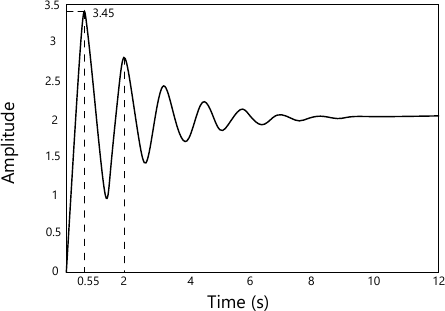MORE IN Mechanical Measurement and Control
MU Mechanical Engineering (Semester 5)
Mechanical Measurement and Control
December 2014
Total marks: --
Total time: --
INSTRUCTIONS
(1) Assume appropriate data and state your reasons
(2) Marks are given to the right of every question
(3) Draw neat diagrams wherever necessary

1 (a) Following table list the measuring instruments (left hand side column of the table) for measuring mechanical properties (right band side column of the table) of the system. Student shall match the measuring instrument with the corresponding mechanical property.
 Measuring Instruments Properties Optical pyrometer Temperature McLeod Gauge Speed Rotameter Pressure Stroboscope Flow rate

Further student shall explain only the working principle of the measurement instrument listed on left hand side column of the table.
4 M
1 (b) Construct the block diagram that combines the following set of equations expressed in the 's' notation (Laplace notation).

1) W=X-Y,
2) V=W-Z
3) Z(S+6)=V(S+2)
4) Y(S2+6S+8)=Z
Given X is the input to the system and Y is the output from the block diagram. Find the transfer function.
6 M

2 (a) Explain the following terms with respect to the measurement system:
i) Threshold and Resolution
ii) Sensitivity and Drift
iii) Hysteresis
5 M
2 (b) With a neat sketch explain the working of LVDT.
5 M
2 (c) Consider the following state representation of single input single output system $\begin{Bmatrix} \cdot \\ x_1\\ \cdot \\ x_2 \\ \cdot \\ x_3 \end{Bmatrix} = \begin{bmatrix} 0 &1 &0 \\0 &0 &1 \\-1 &-3 &-2 \end{bmatrix} \begin{Bmatrix} x_1 \\x_2 \\ x_3 \end{Bmatrix} + \begin{Bmatrix} 0\\0 \\1 \end{Bmatrix} u (t), \\ and \ y(t) = \begin{bmatrix} 1 &0 &0 \end{bmatrix} \begin{Bmatrix} x_1\\x_2 \\x_3 \end{Bmatrix}$ Here x1, x2 and x3 are state-variables, u(t) is a force vector and y(t) being the system response. Obtain transfer function of the system.
10 M

3 (a) With a neat sketch explain the constructional feature and working of (i) piezoelectric accelerometer, (ii) Ionization gauge for pressure measurement.
10 M
3 (b) Figure 1 shows the unit step response of a second order system.
Determine the following from the plot
i) Gain
ii) Damping ratio
iii) Natural Frequency
iv) Transfer function10 M

4 (a) Consider a single strain gauge of resistance 120Ω mounted along the axial direction of an axially loaded specimen of steel (E=200 GPa). If the percentage change in length of the rod due to loading is 3% and the corresponding change in resistivity of the strain gauge material is 0.3%, estimate the percentage change in the resistance of the strain gauge and its gauge factor; Poisson ratio=0.3. If the strain gauge is connected to a measurement device capable of determine change in resistance with an accurate of ± 0.02 Ω, what is the uncertainty in stress that would result in using this resistance measurement device?
10 M
4 (b) For a control system open loop transfer function consist of $G(s) H(s) = \dfrac {K}{S^2 (s+2)(S+3)} .$ Find the value of K" to limit steady state error to 10
10 M

5 (a) For a particular unity feed-back system $G(S) = \dfrac {242 (S+5)} {S(S+1)(S^2+5S+121}$ Sketch a Bode plot. Further comment on stability of the system.
10 M
5 (b) With a neat sketch explain working of an Operational Amplifier (Op-Amp). Enumerate limitations of the same.
10 M

6 (a) Draw the root-locus of the feedback system whose open-loop transfer function is given by $G(S) H(S) = \dfrac {K}{S^2(S+1)}$
10 M
6 (b) Explain generalized measurement system elements with block diagram. Describe its functions with suitable example.
10 M

More question papers from Mechanical Measurement and Control